Скачать презентацию An Introduction to Terra ME Pedro Ribeiro de

1386c3de14dd860900d8e119d2d28b1b.ppt

• Количество слайдов: 30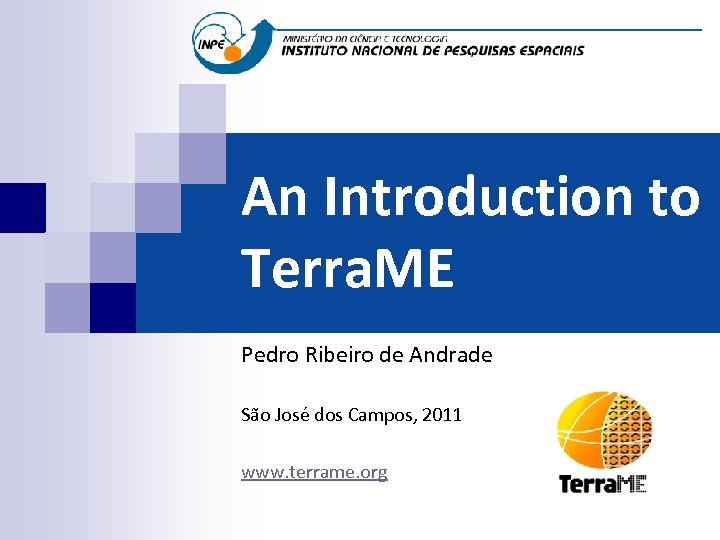An Introduction to Terra. ME Pedro Ribeiro de Andrade São José dos Campos, 2011 www. terrame. org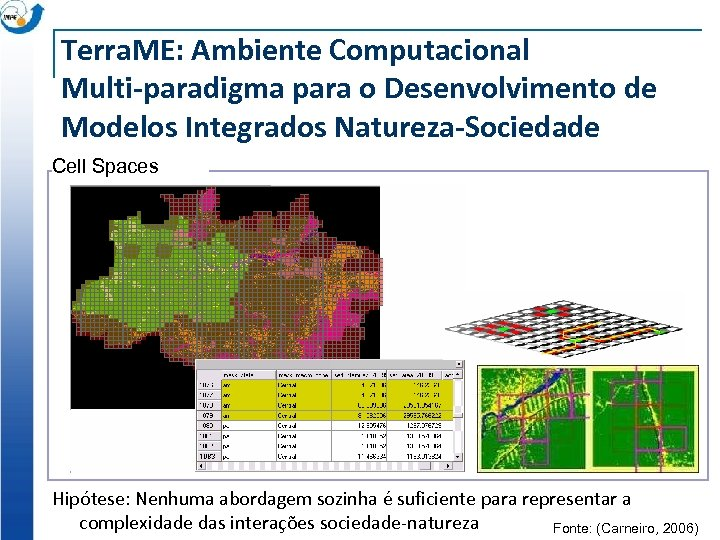Terra. ME: Ambiente Computacional Multi-paradigma para o Desenvolvimento de Modelos Integrados Natureza-Sociedade Cell Spaces Hipótese: Nenhuma abordagem sozinha é suficiente para representar a complexidade das interações sociedade-natureza Fonte: (Carneiro, 2006)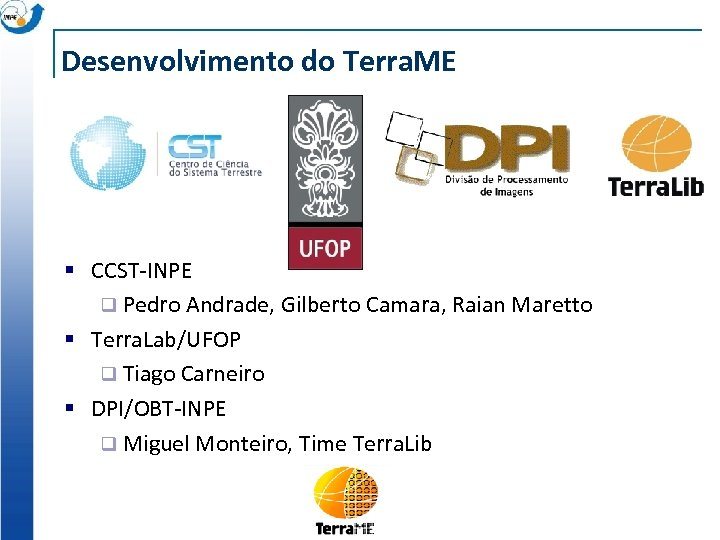Desenvolvimento do Terra. ME § CCST-INPE q Pedro Andrade, Gilberto Camara, Raian Maretto § Terra. Lab/UFOP q Tiago Carneiro § DPI/OBT-INPE q Miguel Monteiro, Time Terra. Lib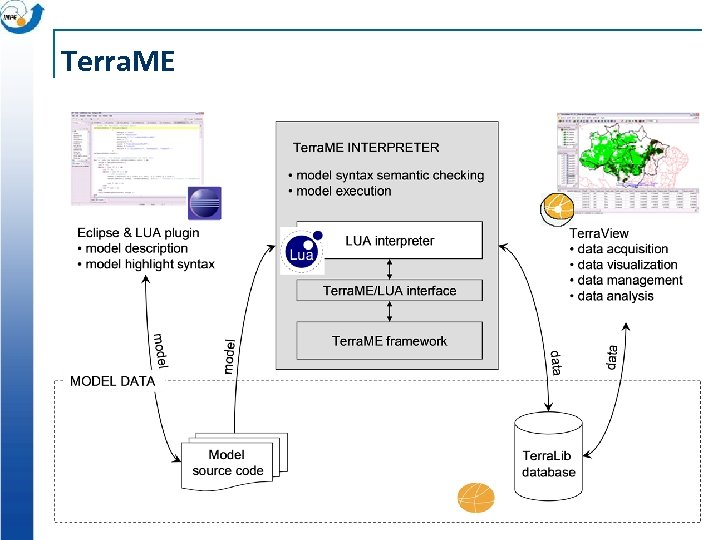Terra. ME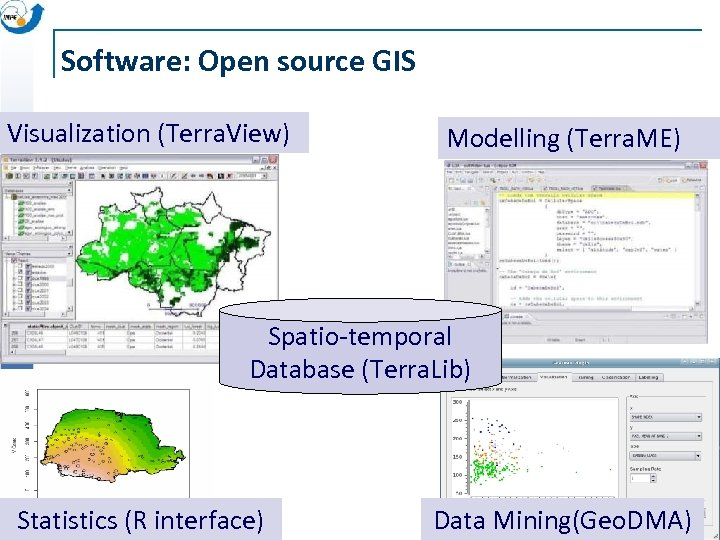Software: Open source GIS Visualization (Terra. View) Modelling (Terra. ME) Spatio-temporal Database (Terra. Lib) Statistics (R interface) Data Mining(Geo. DMA)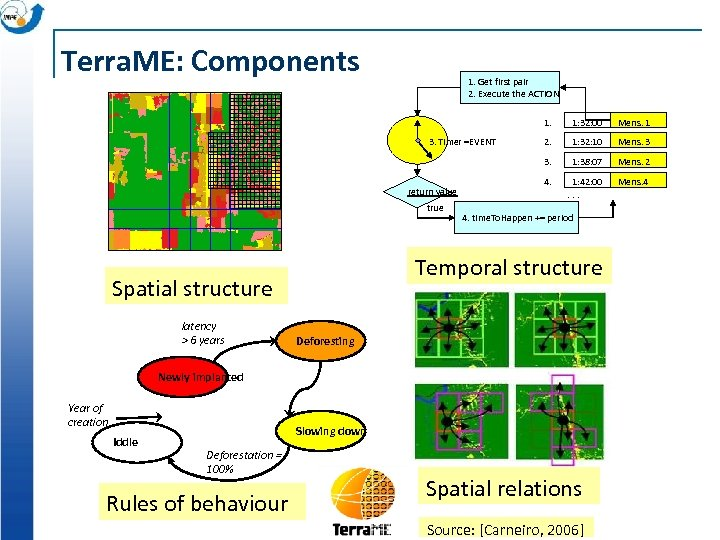Terra. ME: Components 1. Get first pair 2. Execute the ACTION 1. return value true 2. 1: 32: 10 Mens. 3 1: 38: 07 Mens. 2 4. 1: 42: 00. . . Mens. 4 4. time. To. Happen += period Temporal structure Spatial structure latency > 6 years Mens. 1 3. Timer =EVENT 1: 32: 00 Deforesting Newly implanted Year of creation Iddle Slowing down Deforestation = 100% Rules of behaviour Spatial relations Source: [Carneiro, 2006]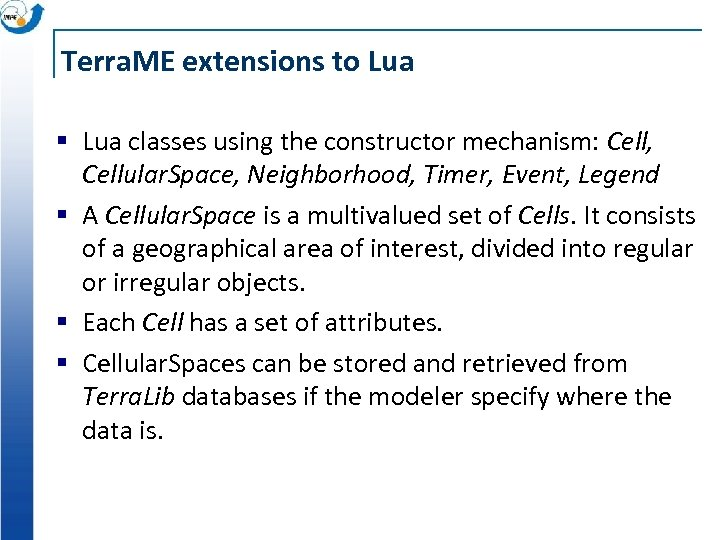Terra. ME extensions to Lua § Lua classes using the constructor mechanism: Cell, Cellular. Space, Neighborhood, Timer, Event, Legend § A Cellular. Space is a multivalued set of Cells. It consists of a geographical area of interest, divided into regular or irregular objects. § Each Cell has a set of attributes. § Cellular. Spaces can be stored and retrieved from Terra. Lib databases if the modeler specify where the data is.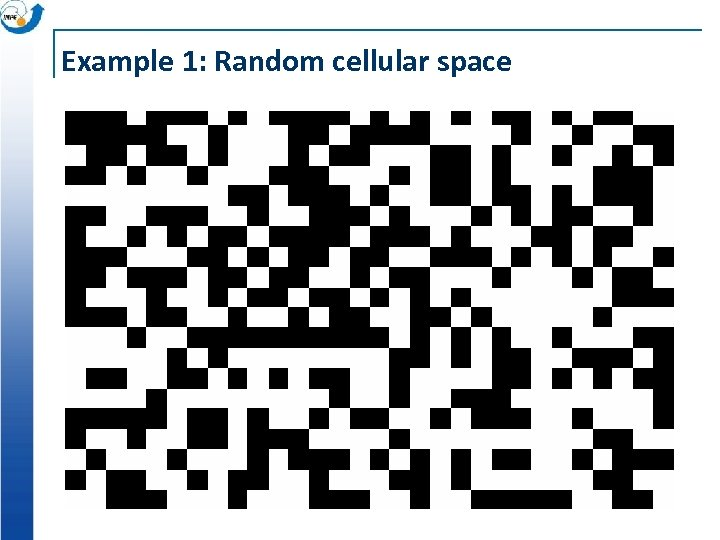Example 1: Random cellular space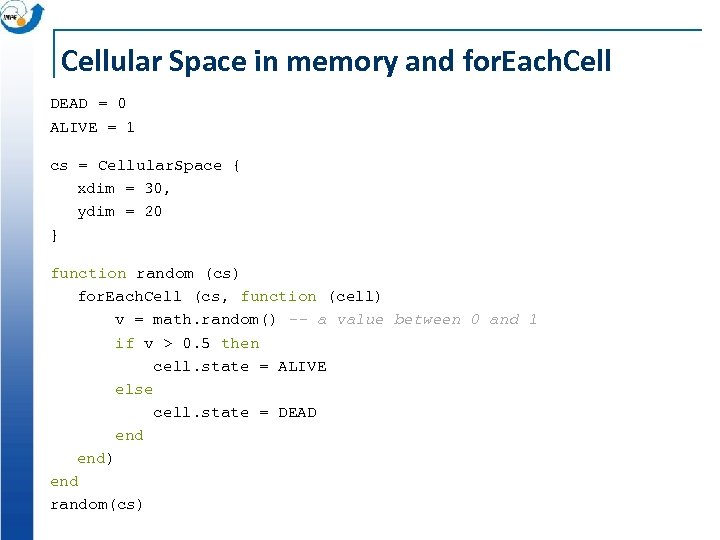Cellular Space in memory and for. Each. Cell DEAD = 0 ALIVE = 1 cs = Cellular. Space { xdim = 30, ydim = 20 } function random (cs) for. Each. Cell (cs, function (cell) v = math. random() -- a value between 0 and 1 if v > 0. 5 then cell. state = ALIVE else cell. state = DEAD end) end random(cs)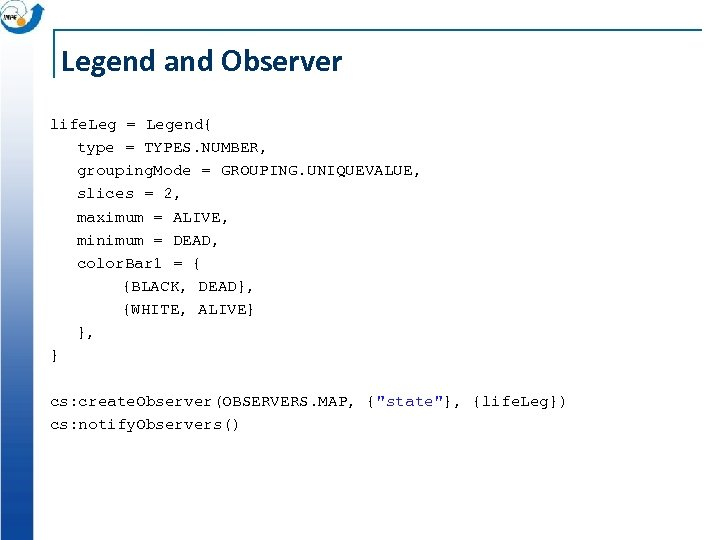Legend and Observer life. Leg = Legend{ type = TYPES. NUMBER, grouping. Mode = GROUPING. UNIQUEVALUE, slices = 2, maximum = ALIVE, minimum = DEAD, color. Bar 1 = { {BLACK, DEAD}, {WHITE, ALIVE} }, } cs: create. Observer(OBSERVERS. MAP, {"state"}, {life. Leg}) cs: notify. Observers()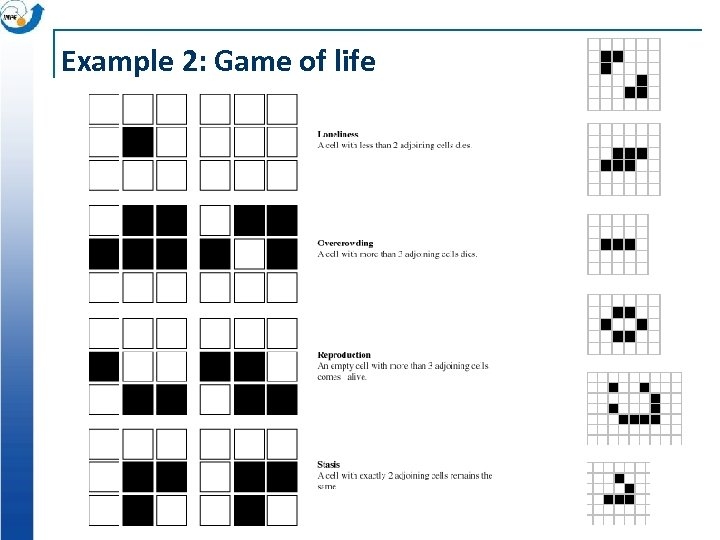Example 2: Game of life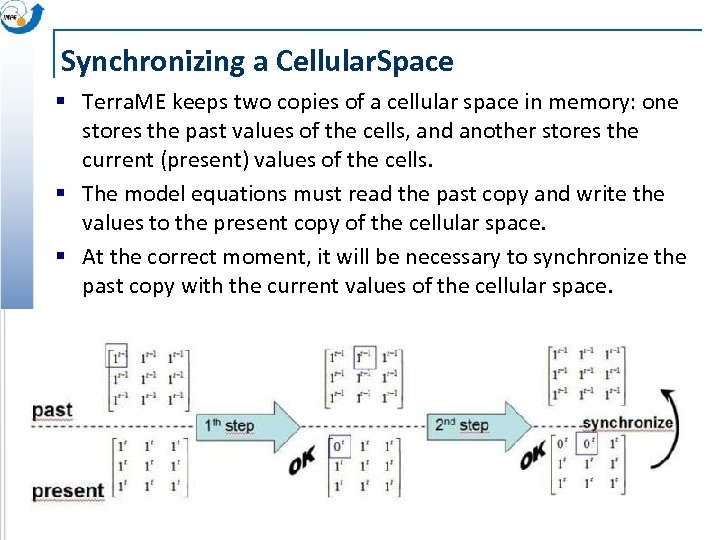Synchronizing a Cellular. Space § Terra. ME keeps two copies of a cellular space in memory: one stores the past values of the cells, and another stores the current (present) values of the cells. § The model equations must read the past copy and write the values to the present copy of the cellular space. § At the correct moment, it will be necessary to synchronize the past copy with the current values of the cellular space.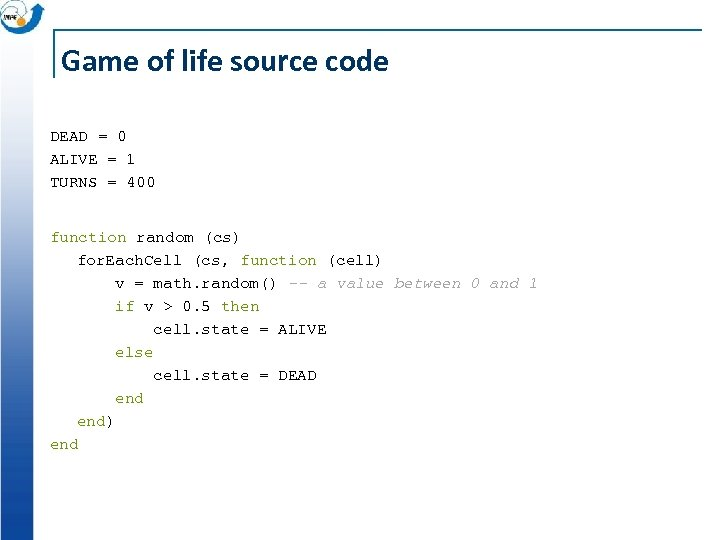Game of life source code DEAD = 0 ALIVE = 1 TURNS = 400 function random (cs) for. Each. Cell (cs, function (cell) v = math. random() -- a value between 0 and 1 if v > 0. 5 then cell. state = ALIVE else cell. state = DEAD end) end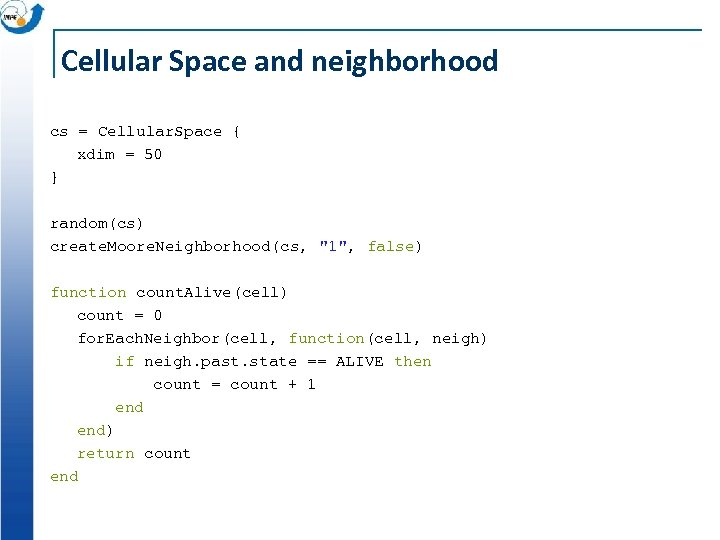Cellular Space and neighborhood cs = Cellular. Space { xdim = 50 } random(cs) create. Moore. Neighborhood(cs, "1", false) function count. Alive(cell) count = 0 for. Each. Neighbor(cell, function(cell, neigh) if neigh. past. state == ALIVE then count = count + 1 end) return count end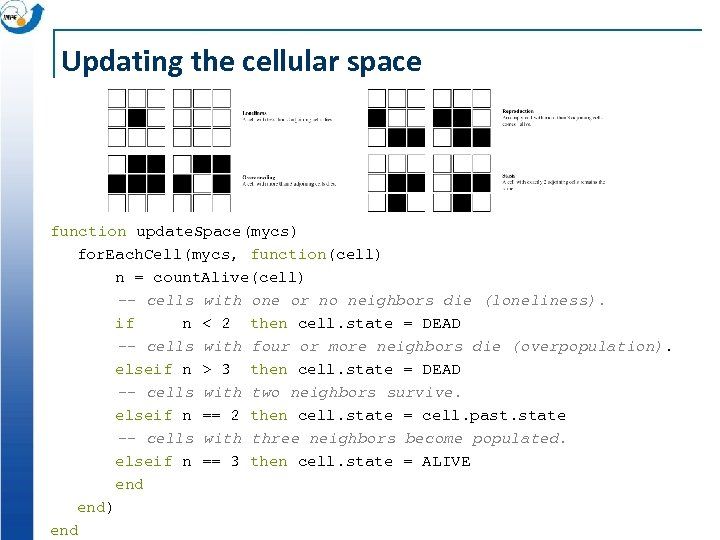Updating the cellular space function update. Space(mycs) for. Each. Cell(mycs, function(cell) n = count. Alive(cell) -- cells with one or no neighbors die (loneliness). if n < 2 then cell. state = DEAD -- cells with four or more neighbors die (overpopulation). elseif n > 3 then cell. state = DEAD -- cells with two neighbors survive. elseif n == 2 then cell. state = cell. past. state -- cells with three neighbors become populated. elseif n == 3 then cell. state = ALIVE end) end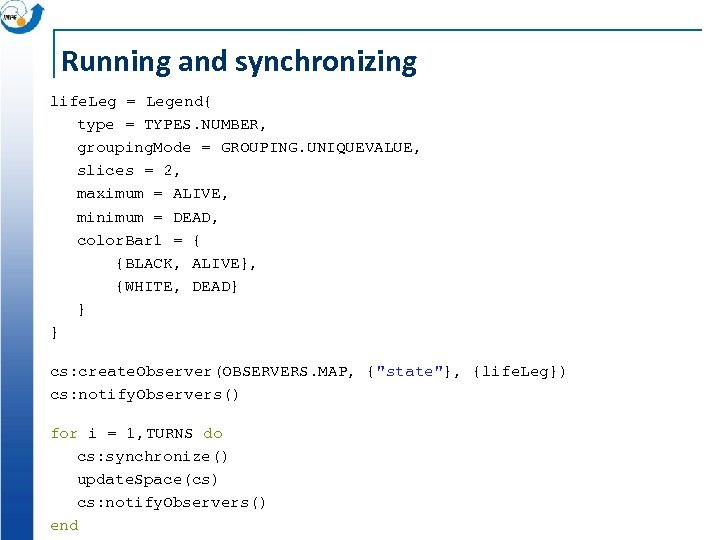Running and synchronizing life. Leg = Legend{ type = TYPES. NUMBER, grouping. Mode = GROUPING. UNIQUEVALUE, slices = 2, maximum = ALIVE, minimum = DEAD, color. Bar 1 = { {BLACK, ALIVE}, {WHITE, DEAD} } } cs: create. Observer(OBSERVERS. MAP, {"state"}, {life. Leg}) cs: notify. Observers() for i = 1, TURNS do cs: synchronize() update. Space(cs) cs: notify. Observers() end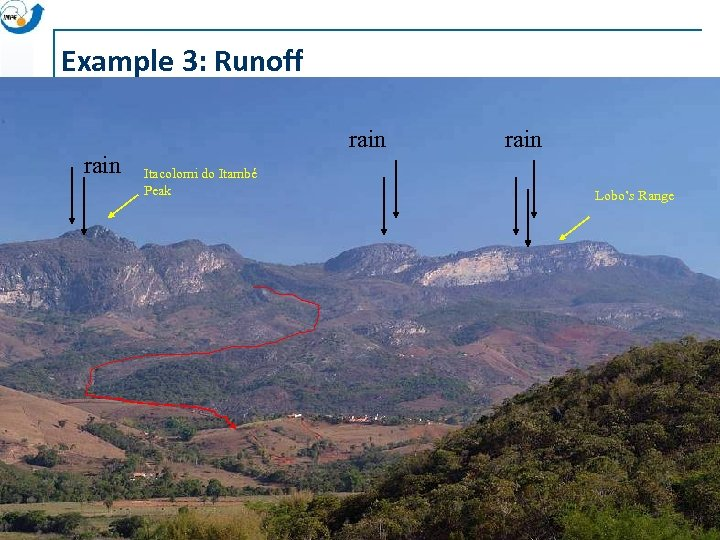Example 3: Runoff rain Itacolomi do Itambé Peak rain Lobo’s Range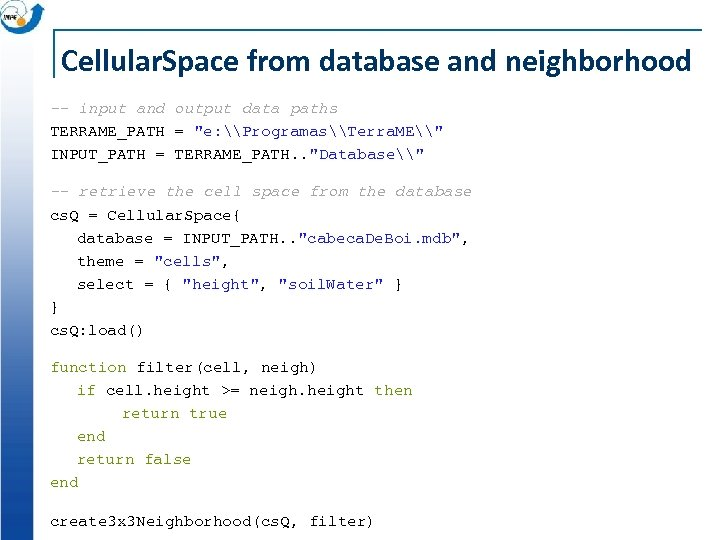Cellular. Space from database and neighborhood -- input and output data paths TERRAME_PATH = "e: \Programas\Terra. ME\" INPUT_PATH = TERRAME_PATH. . "Database\" -- retrieve the cell space from the database cs. Q = Cellular. Space{ database = INPUT_PATH. . "cabeca. De. Boi. mdb", theme = "cells", select = { "height", "soil. Water" } } cs. Q: load() function filter(cell, neigh) if cell. height >= neigh. height then return true end return false end create 3 x 3 Neighborhood(cs. Q, filter)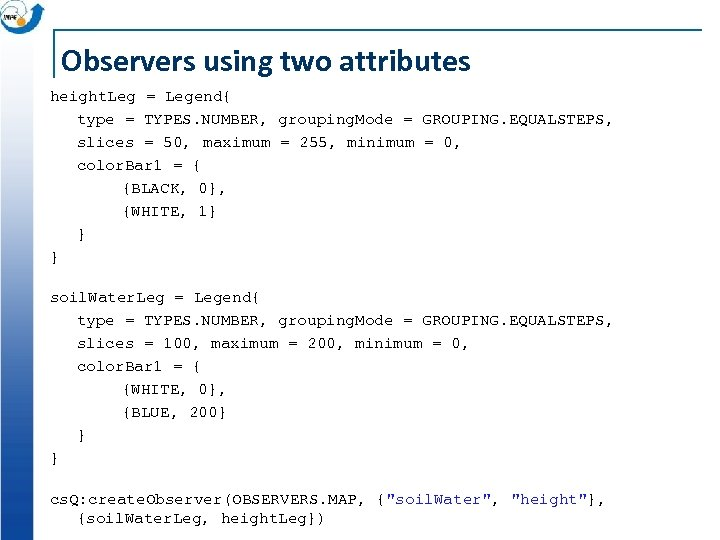Observers using two attributes height. Leg = Legend{ type = TYPES. NUMBER, grouping. Mode = GROUPING. EQUALSTEPS, slices = 50, maximum = 255, minimum = 0, color. Bar 1 = { {BLACK, 0}, {WHITE, 1} } } soil. Water. Leg = Legend{ type = TYPES. NUMBER, grouping. Mode = GROUPING. EQUALSTEPS, slices = 100, maximum = 200, minimum = 0, color. Bar 1 = { {WHITE, 0}, {BLUE, 200} } } cs. Q: create. Observer(OBSERVERS. MAP, {"soil. Water", "height"}, {soil. Water. Leg, height. Leg})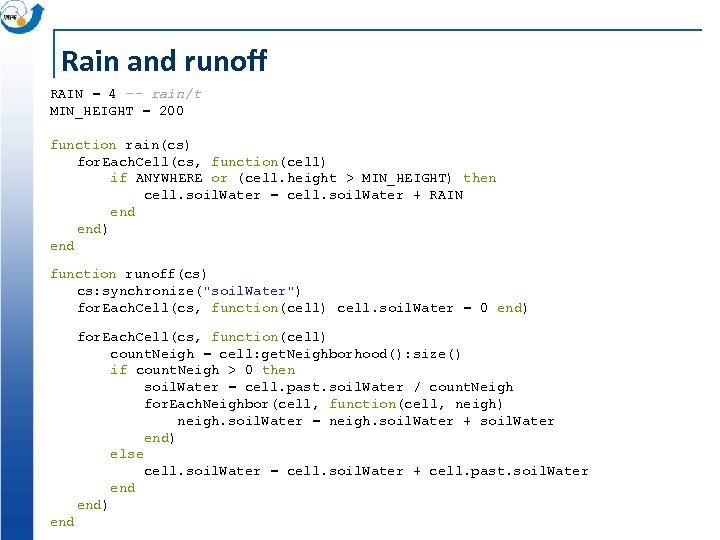Rain and runoff RAIN = 4 -- rain/t MIN_HEIGHT = 200 function rain(cs) for. Each. Cell(cs, function(cell) if ANYWHERE or (cell. height > MIN_HEIGHT) then cell. soil. Water = cell. soil. Water + RAIN end) end function runoff(cs) cs: synchronize("soil. Water") for. Each. Cell(cs, function(cell) cell. soil. Water = 0 end) for. Each. Cell(cs, function(cell) count. Neigh = cell: get. Neighborhood(): size() if count. Neigh > 0 then soil. Water = cell. past. soil. Water / count. Neigh for. Each. Neighbor(cell, function(cell, neigh) neigh. soil. Water = neigh. soil. Water + soil. Water end) else cell. soil. Water = cell. soil. Water + cell. past. soil. Water end) end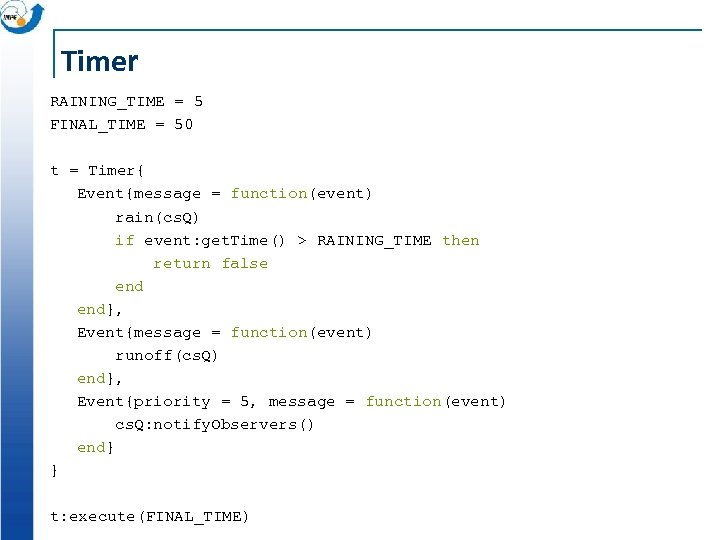Timer RAINING_TIME = 5 FINAL_TIME = 50 t = Timer{ Event{message = function(event) rain(cs. Q) if event: get. Time() > RAINING_TIME then return false end}, Event{message = function(event) runoff(cs. Q) end}, Event{priority = 5, message = function(event) cs. Q: notify. Observers() end} } t: execute(FINAL_TIME)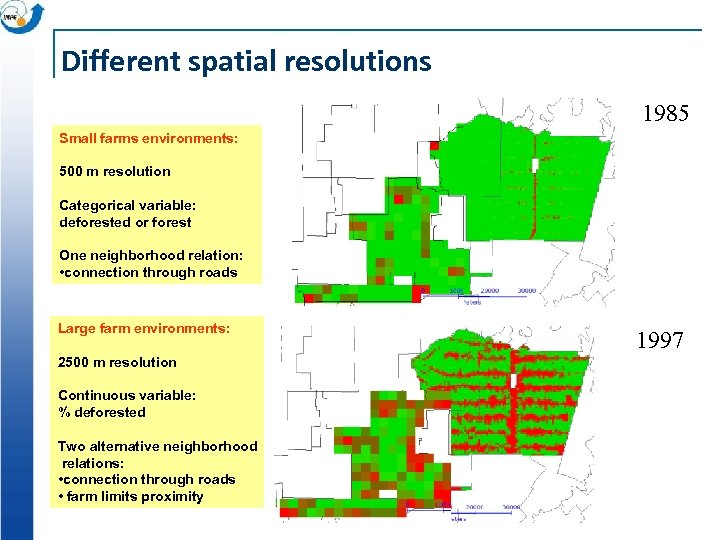Different spatial resolutions 1985 Small farms environments: 500 m resolution Categorical variable: deforested or forest One neighborhood relation: • connection through roads Large farm environments: 2500 m resolution 1997 Continuous variable: % deforested Two alternative neighborhood relations: • connection through roads • farm limits proximity 1997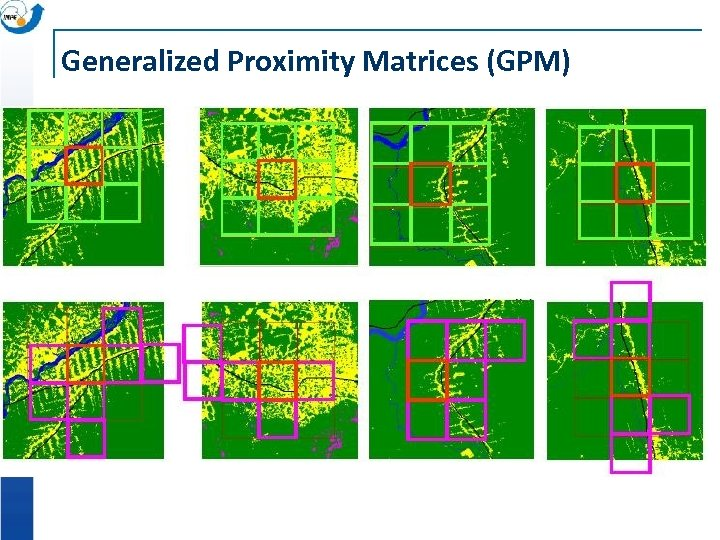Generalized Proximity Matrices (GPM)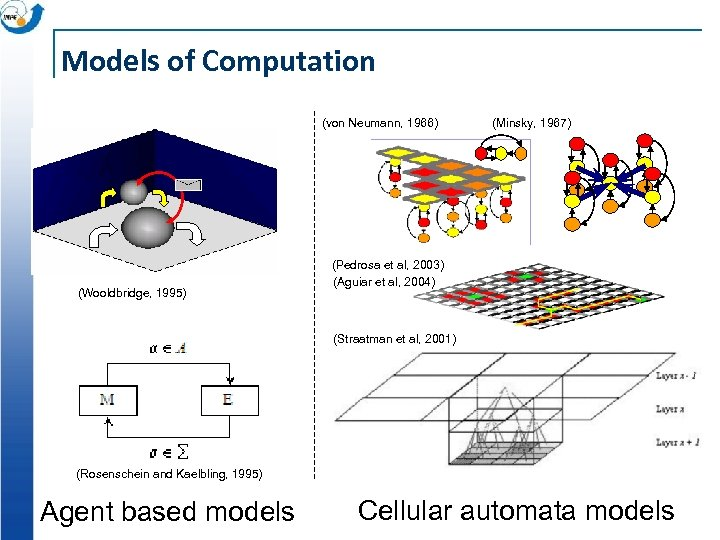Models of Computation (von Neumann, 1966) (Wooldbridge, 1995) (Minsky, 1967) (Pedrosa et al, 2003) (Aguiar et al, 2004) (Straatman et al, 2001) (Rosenschein and Kaelbling, 1995) Agent based models Cellular automata models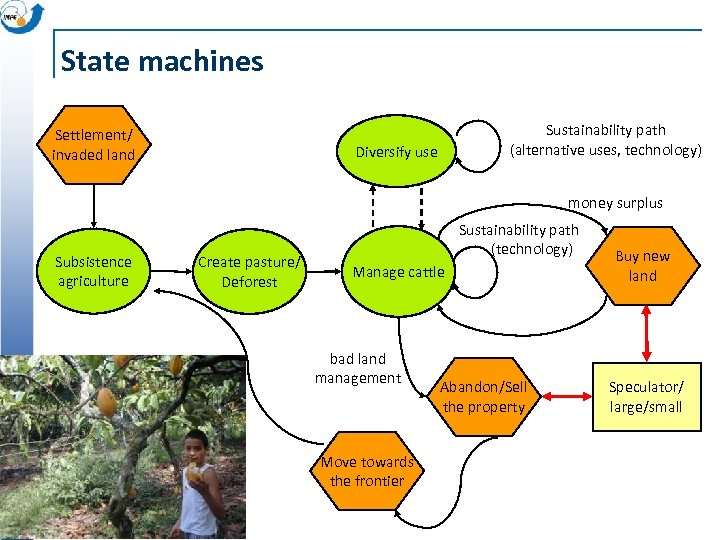State machines Settlement/ invaded land Sustainability path (alternative uses, technology) Diversify use money surplus Subsistence agriculture Create pasture/ Deforest Sustainability path (technology) Manage cattle bad land management Move towards the frontier Abandon/Sell the property Buy new land Speculator/ large/small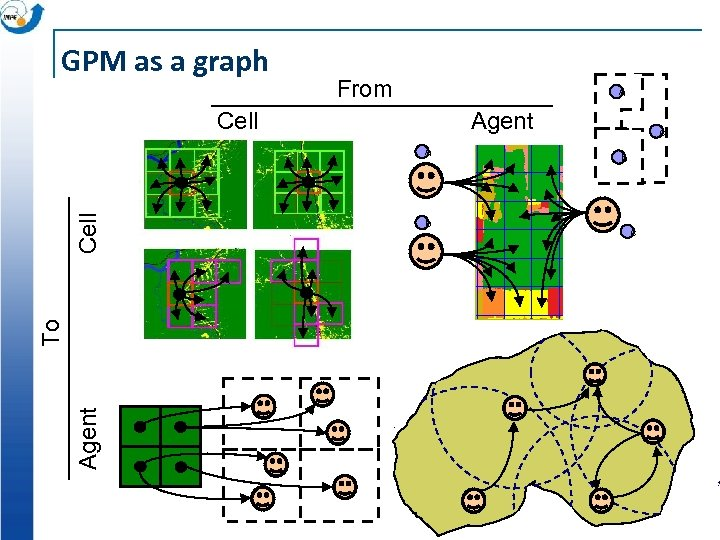GPM as a graph From a Cell Agent To Cell a b c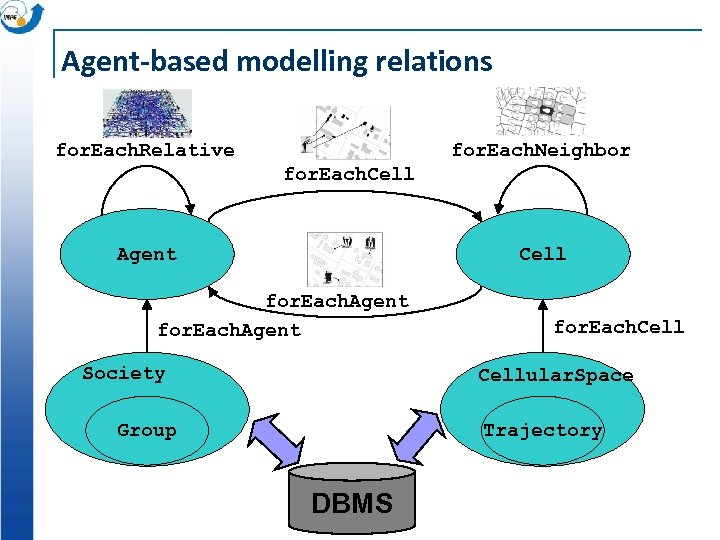Agent-based modelling relations for. Each. Relative for. Each. Neighbor for. Each. Cell Agent Cell for. Each. Agent Society for. Each. Cellular. Space Group Trajectory DBMS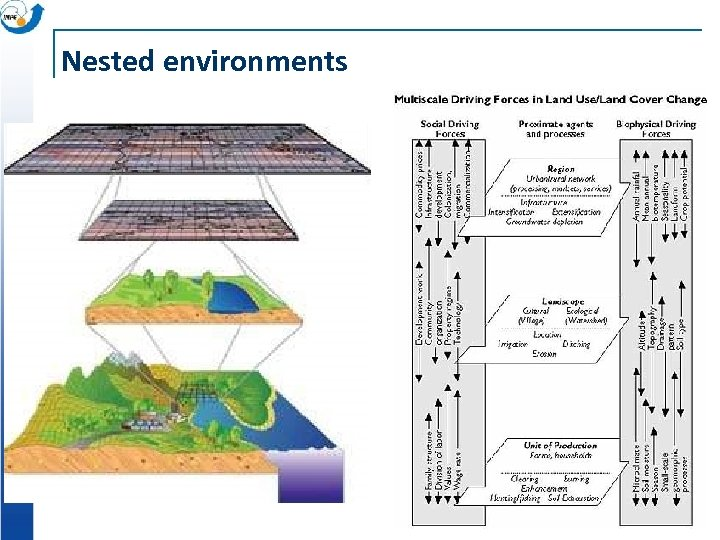Nested environments Prodes/INPE 2000 -2001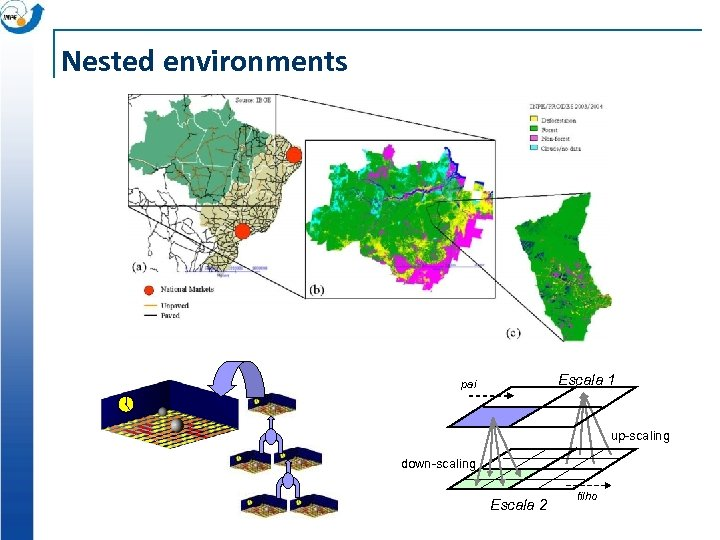Nested environments Escala 1 pai up-scaling down-scaling Escala 2 filhoAn Introduction to Terra. ME Pedro Ribeiro de Andrade São José dos Campos, 2011 www. terrame. org